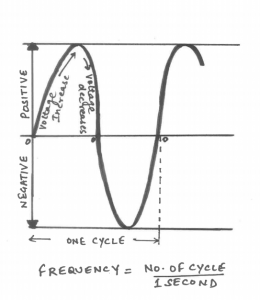Select Page

In the last blog, we learnt about current and voltage. Now, let us learn and discuss different types of current. We know that the flow of electrons constitutes current and voltage is the electric force that causes flow of electrons. For flow of current, electrons must be moving regularly. However, the flow of electrons does not always have to be unidirectional. Current can be classified on the basis of direction of flow of electrons.
Direct Current (D.C):
• If electrons flow constantly in one direction, it is called Direct Current.
• D.C flows along wires in a single direction.
• Cells and battery are the sources of direct current.
• D.C has a positive and a negative terminal.Alternating Current (A.C):
• If the electrons change direction at a regular time interval, the direction of current will also change. Such current is known as Alternating Current.
• Voltage level also changes with current.
• Alternating current is used to deliver power to houses, buildings etc.
• A.C has three terminals Phase, Neutral and Earth.
• A.C moves to and fro. After each period, the current and voltage are back to their original direction and value.
• One oscillation of to and fro movement is called a cycle.
• The number of cycles per second is the frequency of the A.C.
• Frequency is measured in ‘hertz’.
• A.C supply which we get at our homes is 220 volts or 50 hertz.
WHY A.C?
• The devices used for A.C power generation are cheaper and easier to make.
• Current for A.C power can be increased or decreased at a much faster rate.
• D.C power transmission loses a lot of energy.
• Much effort is needed to transmit D.C as compared to A.C over the same distance.
• Hence A.C is the preferred mode of energy across the world.

Share on: